Division with FractionsCustom SearchDIVISION There are two methods commonly used for performing division with fractions. One is the common denominator method and the other is the reciprocal method. Common Denominator Method The common denominator method is an adaptation of the method of like fractions. The rule is as follows: Change the dividend and divisor to like fractions and divide the numerator of the dividend by the numerator of the divisor. This method can be demonstrated with whole numbers, first changing them to fractions with 1 as the denominator. For example, 12 ÷ 4 can be written as follows: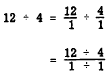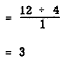If the dividend and divisor are both fractions, as in l/3 divided by l/4, we proceed as follows: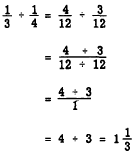Reciprocal Method The word "reciprocal" denotes an interchangeable relationship. It is used in mathematics to describe a specific relationship between two numbers. We say that two numbers are reciprocals of each other if their product is one. In the examplethe fractions 4/1 and 1/4 are reciprocals. Notice the interchangeability: 4 is the reciprocal of 1/4 and 1/4 is the reciprocal of 4. What is the reciprocal of 3/7? It must be a number which, when multiplied by 3/7, produces the product, 1. Therefore,We see that 7/3 is the only number that could fulfill the requirement. Notice that the numerator and denominator of 3/7 were simply interchanged to get its reciprocal. If we know a number, we can always find its reciprocal by dividing 1 by the number. Notice this principle in the following examples: 1. What is the reciprocal of 7?CheckNotice that the cancellation process in this example does not show the usual l’s which result when dividing a number into itself. For example, when 7 cancels 7, the quotient 1 could be shown beside each of the 7’s. However, since 1 as a factor has the same effect whether it is written in or simply understood, the 1’s need not be written. 2. What is the reciprocal of 3/8?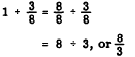Check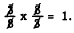3. What is the reciprocal of 5/2? Solution: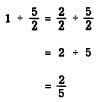Check: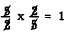4. What is the reciprocal of 3 1/8? SOLUTION: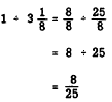Check:The foregoing examples lead to the rule for finding the reciprocal of any number: The reciprocal of a number is the fraction formed when 1 is divided by the number. (If the final result is a whole number, it can be considered as a fraction whose denominator is 1.) A shortcut rule which is purely mechanical and does not involve reasoning may be stated as follows: To find the reciprocal of a number, express the number as a fraction and then invert the fraction. When the numerator of a fraction is 1, the reciprocal is a whole number. The smaller the fraction, the greater is the reciprocal. For example, the reciprocal of 1/1,000 is 1,000. Also, the reciprocal of any whole number is a proper fraction. Thus the reciprocal of 50 is 1/50 Practice problems. Write the reciprocal of each of the following numbers: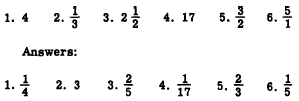The reciprocal method of division makes use of the close association of multiplication and division. In any division problem, we must find the answer to the following question: What number multiplied by the divisor yields the dividend? For example, if the problem is to divide 24 by 6, we must find the factor which, when multiplied by 6, yields 24. Experience tells us that the number we seek is 1/6 of 24. Thus, we may rewrite the problem as follows: Check:In the example 1 1/2 ÷ 3, we could write 3 x ? = 1 1/2. The number we seek must be one-third of 1 1/2. Thus we can do the division by taking one-third of 1 1/2; that is, we multiply 1 1/2 by the reciprocal of 3. Check: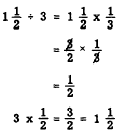Integrated Publishing, Inc. - A (SDVOSB) Service Disabled Veteran Owned Small Business